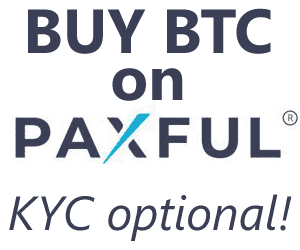Cobinhood to delist these tokens including ETH, OMG, TenX, EOS etcCobinhood has announced on their blog that it will be delisting bucketful of trading pairs of tokens / coins on December 21st 2018, 00:00 UTC +0. Cobinhood says these pairs have weak liquidity and low trading volume, which is why it will be removing them.

Withdrawals and deposits are unaffected and there’s no minimum withdrawing fee required.

Here is the list of trading pairs to be delisted from Cobinhood exchange. “V” means pairs will not be removed. “X” means, it will be removed.

-	BTC	ETH	USDT
BTC	x	x	v
ETH	v	x	v
COB	v	v	x
CMT	v	x	x
REP	x	x	x
EOS	x	x	v
ETHOS	x	x	x
GNT	x	x	x
NGC	x	x	x
OMG	x	x	x
SAN	x	x	x
SPHTX	x	x	x
SNT	x	x	x
PAY	x	x	x
BAT	x	x	x
GTC	x	x	x
TRX	v	x	x
DRGN	x	x	x
ZRX	x	v	x
DGD	x	x	x
CVC	x	x	x
INS	x	x	x
OCN	x	x	x
ICN	x	x	x
MANA	x	x	x
STK	x	x	x
VOISE	x	x	x
FUN	x	x	x
GNO	x	x	x
UTNP	v	v	x
BDG	x	x	x
ENJ	x	x	x
IOST	x	x	x
BRD	x	x	x
BAR	x	x	x
FSN	x	x	x
DADI	x	x	x
NTK	x	x	x
SUB	x	x	x
ABT	x	x	x
REN	x	x	x
WTC	x	x	x
RFR	x	x	x
BEE	x	x	x
MCO	x	x	x
LYM	x	x	x
QTUM	x	x	x
LTC	x	x	v
GTO	x	x	x
FLUZ	x	x	x
LALA	x	v	x
TBAR	x	x	x
BOT	x	x	x
DXT	x	x	x
CGC	x	x	x
BCH	x	x	v
NEO	x	x	v
GAS	v	x	x
SHPING	x	v	x
MFG	x	x	x
ACT	x	x	x
PLY	v	x	x
DENT	x	x	x
MIOTA	x	x	v
FXT	x	v	x
LAP	x	x	x
HQX	x	x	x
MTN	x	x	x
SMT	x	x	x
BOE	x	x	x
BM	x	x	x
COFI	x	x	x
LSTR	x	x	x
SVD	v	x	x
KWATT	v	x	x
DASH	x	x	v
WELL	x	v	x
KICK	x	x	x
ZEC	v	x	x
XMR	v	x	x
XEM	v	x	x
XRP	x	x	v
OSA	x	x	x
TUSD	x	x	v
DAI	x	x	v
PAX	x	x	v
GUSD	x	x	v
XTZ	v	x	x
LSK	v	x	x
VET	v	x	x
VTHO	v	x	x

Cobinhood will be listing Nano on December 14, 2018.Related topics

We are a participant in the Amazon Services LLC Associates Program, an affiliate advertising program designed to provide a means for us to earn fees by linking to Amazon.com and affiliated sites.